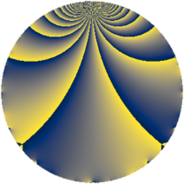# Properties

 Label 1815.2.cLevel $1815$ Weight $2$ Character orbit 1815.c Rep. character $\chi_{1815}(364,\cdot)$ Character field $\Q$ Dimension $108$ Newform subspaces $11$ Sturm bound $528$ Trace bound $6$

# Learn more

## Defining parameters

 Level: $$N$$ $$=$$ $$1815 = 3 \cdot 5 \cdot 11^{2}$$ Weight: $$k$$ $$=$$ $$2$$ Character orbit: $$[\chi]$$ $$=$$ 1815.c (of order $$2$$ and degree $$1$$) Character conductor: $$\operatorname{cond}(\chi)$$ $$=$$ $$5$$ Character field: $$\Q$$ Newform subspaces: $$11$$ Sturm bound: $$528$$ Trace bound: $$6$$ Distinguishing $$T_p$$: $$2$$, $$19$$

## Dimensions

The following table gives the dimensions of various subspaces of $$M_{2}(1815, [\chi])$$.

Total New Old
Modular forms 288 108 180
Cusp forms 240 108 132
Eisenstein series 48 0 48

## Trace form

 $$108q - 108q^{4} - 4q^{5} + 4q^{6} - 108q^{9} + O(q^{10})$$ $$108q - 108q^{4} - 4q^{5} + 4q^{6} - 108q^{9} - 12q^{10} + 24q^{14} + 108q^{16} - 16q^{19} - 4q^{20} + 8q^{21} - 12q^{24} + 4q^{25} + 16q^{26} - 16q^{30} + 24q^{34} + 108q^{36} - 8q^{39} + 28q^{40} + 4q^{45} - 32q^{46} - 108q^{49} - 8q^{50} + 8q^{51} - 4q^{54} - 120q^{56} + 16q^{59} + 20q^{60} + 40q^{61} - 84q^{64} - 40q^{65} + 20q^{70} + 32q^{71} - 32q^{74} - 8q^{75} + 64q^{76} - 32q^{79} + 32q^{80} + 108q^{81} - 40q^{85} + 48q^{86} + 40q^{89} + 12q^{90} - 48q^{94} + 48q^{95} + 28q^{96} + O(q^{100})$$

## Decomposition of $$S_{2}^{\mathrm{new}}(1815, [\chi])$$ into newform subspaces

Label Dim. $$A$$ Field CM Traces $q$-expansion
$$a_2$$ $$a_3$$ $$a_5$$ $$a_7$$
1815.2.c.a $$2$$ $$14.493$$ $$\Q(\sqrt{-1})$$ None $$0$$ $$0$$ $$-2$$ $$0$$ $$q+iq^{2}+iq^{3}+q^{4}+(-1-2i)q^{5}+\cdots$$
1815.2.c.b $$2$$ $$14.493$$ $$\Q(\sqrt{-1})$$ None $$0$$ $$0$$ $$-2$$ $$0$$ $$q+iq^{2}-iq^{3}+q^{4}+(-1+2i)q^{5}+\cdots$$
1815.2.c.c $$4$$ $$14.493$$ $$\Q(i, \sqrt{5})$$ None $$0$$ $$0$$ $$-4$$ $$0$$ $$q-\beta _{2}q^{2}-\beta _{1}q^{3}-3q^{4}+(-1-2\beta _{1}+\cdots)q^{5}+\cdots$$
1815.2.c.d $$6$$ $$14.493$$ 6.0.350464.1 None $$0$$ $$0$$ $$2$$ $$0$$ $$q+(\beta _{3}-\beta _{5})q^{2}-\beta _{3}q^{3}+(-2-\beta _{1}+\cdots)q^{4}+\cdots$$
1815.2.c.e $$6$$ $$14.493$$ 6.0.350464.1 None $$0$$ $$0$$ $$2$$ $$0$$ $$q-\beta _{4}q^{2}-\beta _{3}q^{3}+(-\beta _{1}+\beta _{2})q^{4}+\cdots$$
1815.2.c.f $$8$$ $$14.493$$ 8.0.49787136.1 None $$0$$ $$0$$ $$16$$ $$0$$ $$q+\beta _{6}q^{2}-\beta _{3}q^{3}+\beta _{4}q^{4}+(2-\beta _{3}+\cdots)q^{5}+\cdots$$
1815.2.c.g $$8$$ $$14.493$$ $$\Q(\zeta_{24})$$ None $$0$$ $$0$$ $$-8$$ $$0$$ $$q+(-\zeta_{24}+\zeta_{24}^{5})q^{2}+\zeta_{24}^{3}q^{3}+(-1+\cdots)q^{5}+\cdots$$
1815.2.c.h $$12$$ $$14.493$$ $$\mathbb{Q}[x]/(x^{12} + \cdots)$$ None $$0$$ $$0$$ $$-2$$ $$0$$ $$q+\beta _{1}q^{2}+\beta _{4}q^{3}+(-2+\beta _{2})q^{4}-\beta _{6}q^{5}+\cdots$$
1815.2.c.i $$12$$ $$14.493$$ $$\mathbb{Q}[x]/(x^{12} + \cdots)$$ None $$0$$ $$0$$ $$-2$$ $$0$$ $$q+\beta _{1}q^{2}-\beta _{4}q^{3}+(-2+\beta _{2})q^{4}-\beta _{5}q^{5}+\cdots$$
1815.2.c.j $$24$$ $$14.493$$ None $$0$$ $$0$$ $$-2$$ $$0$$
1815.2.c.k $$24$$ $$14.493$$ None $$0$$ $$0$$ $$-2$$ $$0$$

## Decomposition of $$S_{2}^{\mathrm{old}}(1815, [\chi])$$ into lower level spaces

$$S_{2}^{\mathrm{old}}(1815, [\chi]) \cong$$ $$S_{2}^{\mathrm{new}}(55, [\chi])$$$$^{\oplus 4}$$$$\oplus$$$$S_{2}^{\mathrm{new}}(165, [\chi])$$$$^{\oplus 2}$$$$\oplus$$$$S_{2}^{\mathrm{new}}(605, [\chi])$$$$^{\oplus 2}$$